# The Triangular Prism

The triangular prism is a simple 3D uniform polyhedron bounded by 5 polygons (2 triangles and 3 squares), 9 edges, and 6 vertices. It may be considered to be the extrusion of the triangle.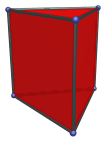Two triangular prisms can be glued together at their square faces in gyro orientation to produce a gyrobifastigium (J26), a Johnson solid: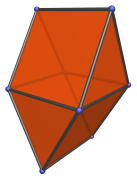Besides the obvious construction as the extrusion of the triangle, the triangular prism may also be considered as a digonal cupola: just as the triangular cupola can be constructed as the convex hull of a triangle and a Stott-expanded triangle (hexagon) on parallel planes, so the triangular prism can be constructed as the convex hull of a digon and a Stott-expanded digon (square) on parallel planes. Thus, the gyrobifastigium may be considered to be a digonal gyrobicupola, which explains its place as the 26th Johnson solid, the first among the bicupolae.

The triangular prism can also be augmented by up to 3 square pyramids, forming the augmented triangular prism (J49), the biaugmented triangular prism (J50), and the triaugmented triangular prism (J51).

## Projections

In order to be able to identify the triangular prism in various projections of 4D objects, it is useful to know how it appears from various viewpoints. The following are some of the commonly-encountered views:

Projection Envelope Description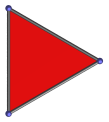Triangle

Parallel projection centered on a triangular face.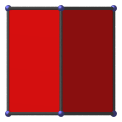Square

Parallel projection centered on a vertical edge.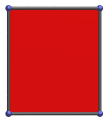Rectangle

Parallel projection parallel to a square face. The left edge of the projection is the image of a square face.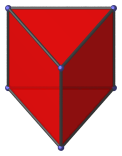Non-uniform pentagon

Vertex-centered parallel projection.

## Coordinates

The Cartesian coordinates of the triangular prism, centered on the origin and having edge length 2, are:

• (−1, −1/√3, ±1)
• (1, −1/√3, ±1)
• (0, 2/√3, ±1)

## Occurrences

The triangular prism is a very versatile polyhedron that occurs in many 4D uniform polytopes, including:

It also occurs in many CRF polychora, including (but not limited to):

Last updated 17 Jun 2019.